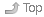# 1 First Midterm TestIndexCalculators are not permitted during the first midterm test. You will benefit from knowing the powers of two from 2^(-4) to 2^16 and the decimal and binary values of the hexadecimal digits from zero to fifteen. Of course you can work them out; however, having at least some of them memorized will make things go faster for you on the test. (Remember that hexadecimal “A” = decimal 10 = binary 1010.)

# 2 Lecture Notes for This WeekIndex## 2.2 From Blackboard Course DocumentsIndexThese documents have restricted distribution and cannot be put on the Course Home Page.

• 01.ppt - Introduction (vonNeumann, prefixes, reciprocal)
• 02.ppt - Data Representation
• omit “Booth’s algorithm” slides 50-52
• ignore slides 63-70 (the “simplified” model)
• slide 72 is wrong: IEEE 754 +0.0 is equal to -0.0
• ignore slides 75-76 (floating-point multiplication)
• use my web pages and assignments instead for floating-point info
• you do not need to know how to do math with sign-magnitude numbers
• you do need to know how to convert them to/from decimal
• you do not need to know how to do math with one’s complement numbers
• you do need to know how to convert them to/from decimal
• you need to know how to do addition with two’s complement numbers
• you also need to know how to convert them to/from decimal
• you do not have to multiply or divide or subtract any binary numbers
• omit most of the math in 2.8 “Error Detection and Correction”
• omit slides 94-100
• omit slides 103-115

## 2.4 From the Classroom Whiteboard/ChalkboardIndex• Your in-class notes go here.
``````Author:
| Ian! D. Allen  -  idallen@idallen.ca  -  Ottawa, Ontario, Canada